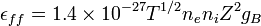# Bremsstrahlung

Bremsstrahlung is a German word for brake radiation. It is the electromagnetic radiation emitted when charged particles are brought to a stop or when they change their velocity in other ways. The term was originally used to describe the spectrum of X-ray tubes, but it also applies to plasma in space.

## In astrophysics

The dominant, luminous component in a cluster of galaxies is the 107 to 108 K intracluster medium (ICM). The emission from the ICM is characterized by thermal Bremsstrahlung. Thermal Bremsstrahlung radiation is when the particles populating the emitting plasma are at a uniform temperature and are distributed according to the Maxwell–Boltzmann distribution$f (v) = 4 \pi \left(\frac{m}{2 \pi kT}\right)^{3/2} v^2 \exp \left[ \frac{-mv^2}{2kT} \right]$

where speed, v, is defined as$v = \sqrt{v_x^2 + v_y^2 + v_z^2}.$

The bulk emission from this gas is thermal Bremsstrahlung. The power emitted per cubic centimeter per second can be written in the compact form$\epsilon_{ff} = 1.4\times 10^{-27} T^{1/2} n_{e} n_{i} Z^{2} g_B$

with cgs units [erg cm-3 s-1] and where 'ff' stands for free-free, 1.4x10-27 is the condensed form of the physical constants and geometrical constants associated with integrating over the power per unit area per unit frequency, ne and ni are the electron and ion densities, respectively, Z is the number of protons of the bending charge, gB is the frequency averaged Gaunt factor and is of order unity, and T is the global x-ray temperature determined from the spectral cut-off frequency$\hbar\nu=kT$

below which no photons are created because the energy supplied by acceleration of the electron by the positively charged nucleus is less than the minimum energy required for creation of a photon.

This process is also known as Bremsstrahlung cooling since the plasma is optically thin to photons at these energies and the energy radiated is emitted freely into the Universe.

This radiation is in the energy range of X-Rays and can be easily observed with space-based telescopes such as Chandra X-Ray Observatory, XMM-Newton, ROSAT, ASCA, EXOSAT, Astro-E2, and future missions like Con-X and NeXT.Some content on this page may previously have appeared on Citizendium.